# Binary Tree Insertion in Rust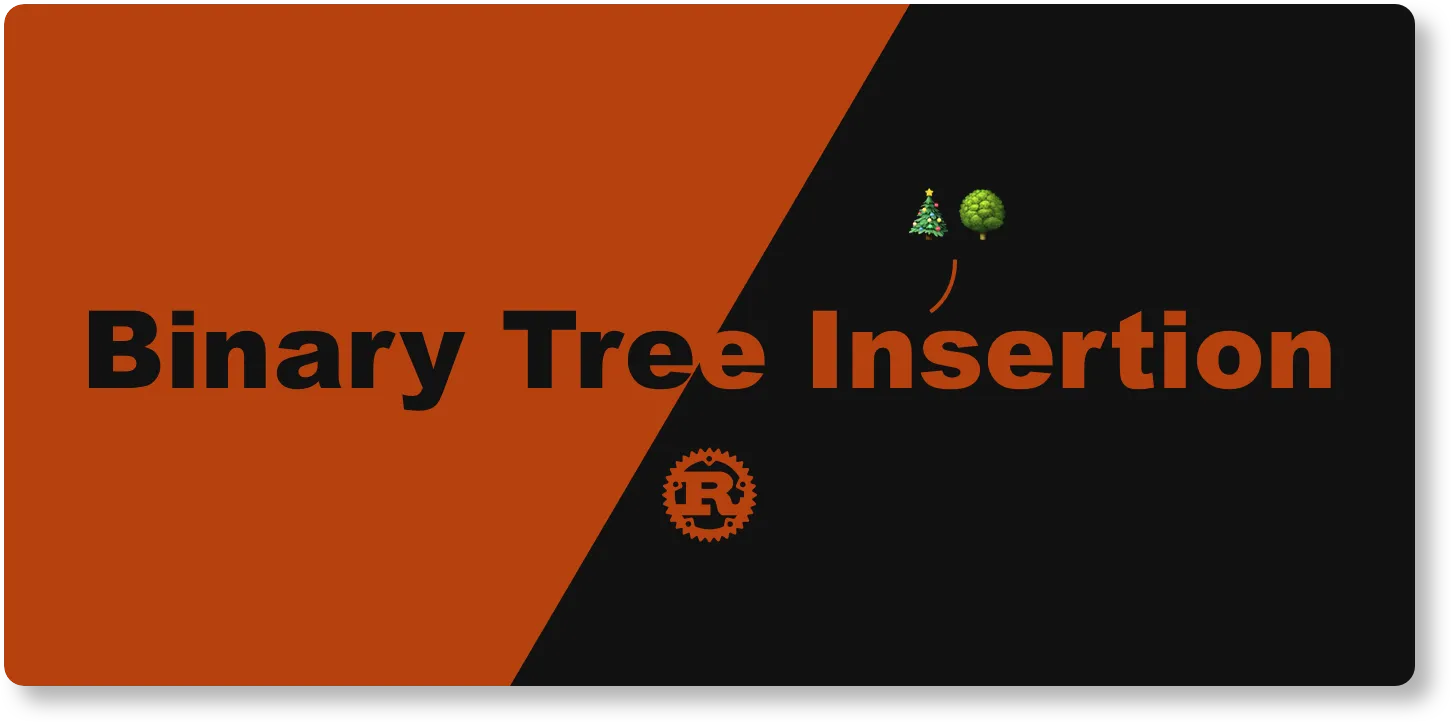## TL;DR

• 🌳 We'll implement a Binary Tree together.
• 🌾 We'll discuss a couple of ways to insert a node in a Binary Tree.
• 🔬 We'll discuss Rust's ownership in action.
• We'll touch on more features and syntax in Rust.

I was struggling hard with Rust's ownership when implementing a Binary Tree so I pivoted and re-read about it. After taking my time understanding it and refactoring my code, I finally made a breakthrough😎 I'm very excited to share with you the awesome features in Rust I came across. You'll see interesting concepts like smart pointers and ownership.

Let's get it!

## Data Structure

A Binary Tree data structure look like this: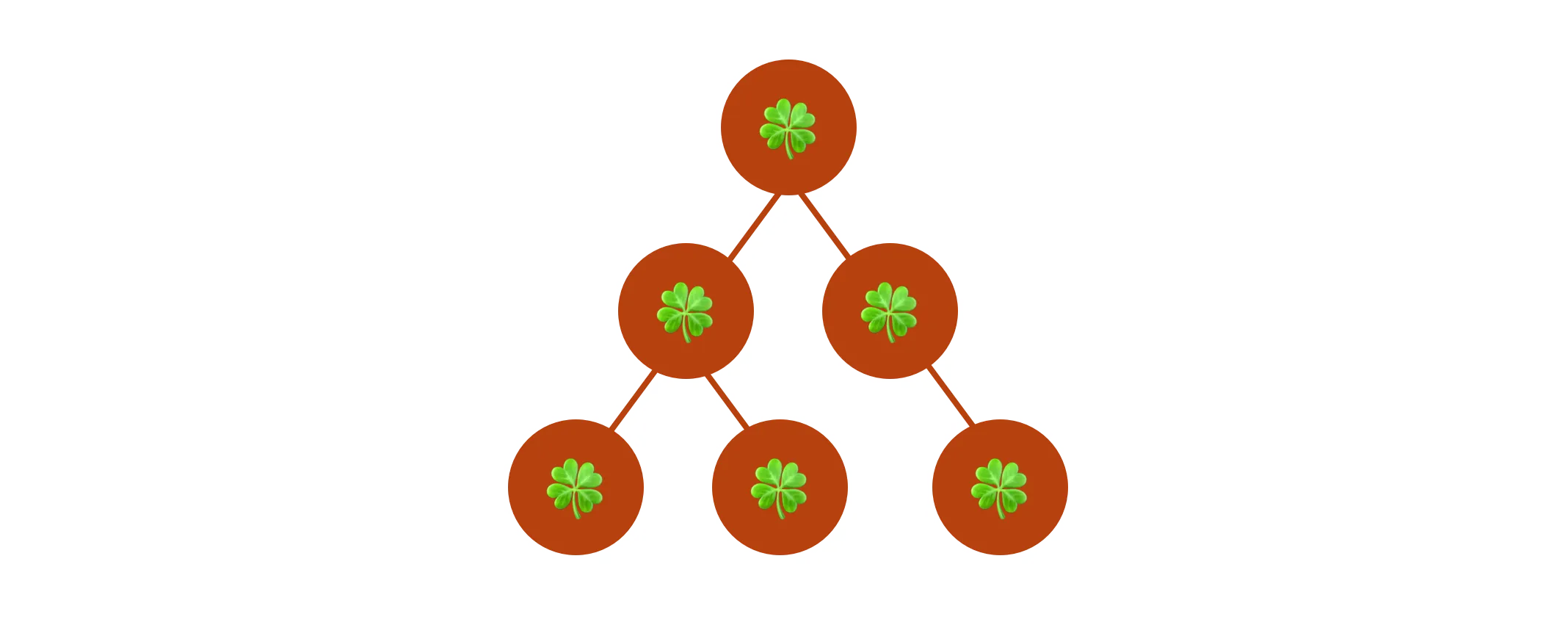Each node has no more than two child nodes. We refer them as `left` child and `right` child. We can translate the description into Rust code like this:

``````pub struct BinaryTree<T> {
pub value: T,
pub left: Option<Box<BinaryTree<T>>>,
pub right: Option<Box<BinaryTree<T>>>,
}
``````

The `BinaryTree` struct holds a value with the generic type `T`. We use `Option` enum to represent that the `left` and `right` child are both optional.

A `Option<T>` is either a `Some` that contains a value of the type `T`, or a `None` that represents it doesn't. Because we are using `Option` to express whether a value is either something or nothing, the Rust compiler can check if we handle all the cases to prevent bugs. Comparing to JavaScript where we use `null` value to express the same concept, `Option` encourages me to handle use cases upfront and it saves me a lot of trouble in runtime.

The `Box` is one of the smart pointers. It saves an address that points to a data in memory. `Box` helps us to create a `BinaryTree` struct with an unknown size, so that we can grow the Binary Tree by inserting nodes without thinking ahead how many nodes we'll have when creating the tree.

Memory management is one of the reason Rust is so performing and interesting to learn.

## Insertion

Before inserting a new Binary Tree node, we need to create an root. Let's implement it:

``````impl<T> BinaryTree<T> {
pub fn new(value: T) -> Self {
BinaryTree {
value,
left: None,
right: None,
}
}
}
``````

The `new` associated function takes the value of `T` and return a `BinaryTree` that holds the value and no child nodes.

Now that we can use `BinaryTree::new` to create a root node, we can think about how to insert child nodes. Intuitively, it would be great if we can insert left or right child node by calling methods on the root node instance. Like this:

``````BinaryTree::new(1)
.left(BinaryTree::new(2))
.right(BinaryTree::new(3))
``````

Luckily, I found an great article from my friend at trivago, Matthias, explaining exactly how to implement it.

``````impl<T> BinaryTree<T> {
pub fn new(value: T) -> Self {
BinaryTree {
value,
left: None,
right: None,
}
}

pub fn left(mut self, node: BinaryTree<T>) -> Self {
self.left = Some(Box::new(node));
self
}

pub fn right(mut self, node: BinaryTree<T>) -> Self {
self.right = Some(Box::new(node));
self
}
}
``````

Now let's write some tests to make sure the associated functions work:

``````#[cfg(test)]
mod tests {
use super::*;

#[test]
fn create_new_tree() {
let tree = BinaryTree::new(1);

assert_eq!(tree.value, 1);
}

#[test]
fn insert_left() {
let tree = BinaryTree::new(1).left(BinaryTree::new(2));

if let Some(node) = tree.left {
assert_eq!(node.value, 2);
}

assert_eq!(tree.right, None);
}

#[test]
fn insert_right() {
let tree = BinaryTree::new(1).right(BinaryTree::new(2));

if let Some(node) = tree.right {
assert_eq!(node.value, 2);
}

assert_eq!(tree.left, None);
}
}
``````

The insertion methods are very flexible and we can easily create a tree in just a few lines:

``````BinaryTree::new(1)
.left(
BinaryTree::new(2)
.left(BinaryTree::new(4))
.right(BinaryTree::new(5))
)
.right(BinaryTree::new(3))
``````

The code creates a Binary Tree like this:It got me thinking.

If I just want to create a balanced Binary Tree without any other requirements, can I insert a node and the tree finds the next available spot for me?

Something like this:

``````let mut root = BinaryTree::new(1);
root.insert(2);
root.insert(3);
root.insert(4);
root.insert(5);
``````

and it creates the same tree structure as we saw above.

We can achieve it by traverse the tree in the breadth-first fashion and insert a node as soon as we found an absent child node.

The easiest way to implement a breath-first traversal is with a Queue. Rust has a `VecDequeue` in the standard library we can use.

``````pub fn insert(&mut self, new_value: T) {
let mut queue: VecDeque<&mut BinaryTree<T>> = VecDeque::new();
queue.push_front(self);

loop {
let BinaryTree {
ref mut left,
ref mut right,
..
} = queue.pop_back().unwrap();

match left {
Some(node) => {
queue.push_front(node);
}
None => {
*left = Some(Box::new(BinaryTree::new(new_value)));
return;
}
}

match right {
Some(node) => {
queue.push_front(node);
}
None => {
*right = Some(Box::new(BinaryTree::new(new_value)));
return;
}
}
}
}
``````

The algorithm is to force the loop to visit sibling nodes first, from left to right, before visiting the next layer of child nodes. In each iteration, we'll check if either the `left` or `right` child is absent. If we found one, that's the next available spot for the new node.

It's a rather straightforward algorithm but I struggled to get it right. The problem was that I didn't understand ownership in Rust.

Let's go through the `insert` method together.

First thing we want to decide is how do we want to use the first argument `self`. `self` is referring to the `BinaryTree` instance the method is called on. What we need from `self` is to be able to mutate the `left` and `right` child node so that we can insert a new node. Simply passing in a mutable reference `&mut self` will do the job because the method don't need to take ownership of `self`.

For the `VecDeque` item data type, we can use the same data type as `self` to store mutable references of `BinaryTree`.

When popping the queue inside the loop, we want to use a mutable reference to `left` and `right` because we want to insert the new node.

When inserting the new node, we dereference the `left` and `right` to allow the new node assignment, like this: `*left = Some(Box::new(BinaryTree::new(new_value)))`.

It took me some time to figure out how to borrow or move the data in the method. Once I got it, it make so much sense!

Let's write some tests for it🔬

``````#[test]
fn insert() {
let mut tree = BinaryTree::new(1);
tree.insert(2);
tree.insert(3);
tree.insert(4);
tree.insert(5);

assert_eq!(
tree,
BinaryTree::new(1)
.left(
BinaryTree::new(2)
.left(BinaryTree::new(4))
.right(BinaryTree::new(5))
)
.right(BinaryTree::new(3))
);

tree.insert(6);

assert_eq!(
tree,
BinaryTree::new(1)
.left(
BinaryTree::new(2)
.left(BinaryTree::new(4))
.right(BinaryTree::new(5))
)
.right(BinaryTree::new(3).left(BinaryTree::new(6)))
)
}
``````

If we run the tests, you'll see a error message like this: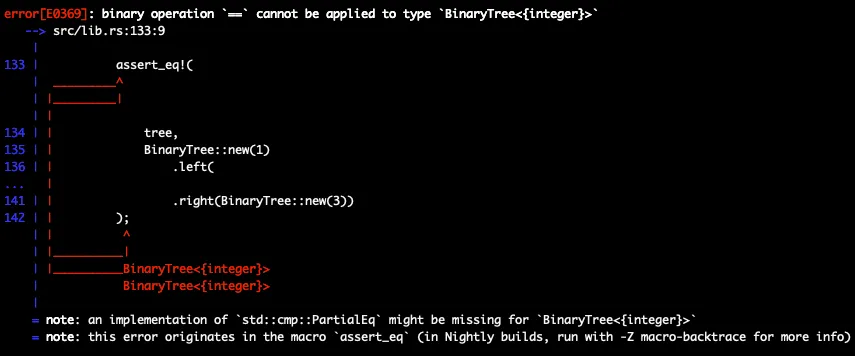It's because the trees couldn't compare with each other. We can fix it by adding `PartialEq` trait to the `BinaryTree` struct.

``````+ #[derive(PartialEq)]
pub struct BinaryTree<T> {
pub value: T,
pub left: Option<Box<BinaryTree<T>>>,
pub right: Option<Box<BinaryTree<T>>>,
}
``````

## Converting Array into Binary Tree

Now that we have an automatic insertion with the `insert` method, we can consider creating a balanced tree in a more convenient way. For example, I want to have something similar to `Vec::from`: an associated function `BinaryTree::from` that takes in an array and returns a balanced `BinaryTree`.

Let's write a test to visualize the use case better:

``````#[test]
fn create_new_tree_with_from() {
// `BinaryTree::from` takes in a reference of an array because borrowing is sufficient
let tree = BinaryTree::from(&[1, 2, 3, 4, 5, 6]);

assert_eq!(
tree,
BinaryTree::new(1)
.left(
BinaryTree::new(2)
.left(BinaryTree::new(4))
.right(BinaryTree::new(5))
)
.right(BinaryTree::new(3).left(BinaryTree::new(6)))
)
}
``````

To implement `BinaryTree::from`, we can simply iterate through the array and using the `insert` method to create the tree structure.

``````impl<T> BinaryTree<T> {
pub fn from(new_values: &[T]) -> Self {
let (first, rest) = new_values.split_first().unwrap();
let mut root: BinaryTree<T> = BinaryTree::new(*first);

for value in rest {
root.insert(*value)
}
root
}
}
``````

In the function, we created a root node from the first array element and then insert the rest element in the tree one by one.

If you try to test it, you'll see an error message like this: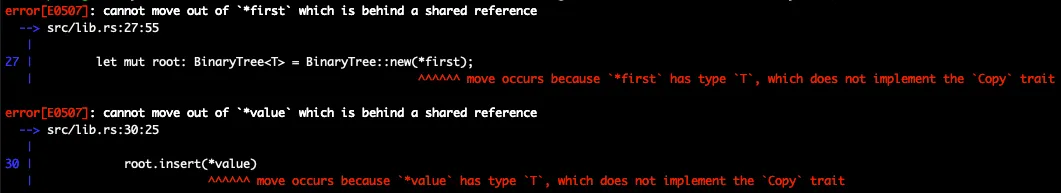We can fix it by specifying the type `T` implements the `Copy` trait.

``````- impl<T> BinaryTree<T> {
+ impl<T> BinaryTree<T>
+ where
+     T: Copy,
+ {
``````

The reason is that the `insert` method actually take ownership over `new_value`. In order to keep the program memory save, the compiler didn't allow us to "move" the array elements into the `insert` method because the array might be referenced in other parts of the program. So what we can do is to pass in a copy (duplicate) of an array element.

Now it should work!

## Final Thought

That's it! The full implementation of our Binary Tree assertion is here⬇️

``````#[derive(Debug, PartialEq)]
pub struct BinaryTree<T> {
pub value: T,
pub left: Option<Box<BinaryTree<T>>>,
pub right: Option<Box<BinaryTree<T>>>,
}

impl<T> BinaryTree<T>
where
T: Copy,
{
///
/// Create a new Binary Tree node.
///
pub fn new(value: T) -> Self {
BinaryTree {
value,
left: None,
right: None,
}
}

///
/// Create a balanced tree from an array reference.
///
pub fn from(new_values: &[T]) -> Self {
let (first, rest) = new_values.split_first().unwrap();
let mut root: BinaryTree<T> = BinaryTree::new(*first);

for value in rest {
root.insert(*value)
}
root
}

///
/// Insert a tree node in the next available branch with breadth first traversal.
///
pub fn insert(&mut self, new_value: T) {
let mut queue: VecDeque<&mut BinaryTree<T>> = VecDeque::new();
queue.push_front(self);
loop {
let BinaryTree {
ref mut left,
ref mut right,
..
} = queue.pop_back().unwrap();

match left {
Some(node) => {
queue.push_front(node);
}
None => {
*left = Some(Box::new(BinaryTree::new(new_value)));
return;
}
}

match right {
Some(node) => {
queue.push_front(node);
}
None => {
*right = Some(Box::new(BinaryTree::new(new_value)));
return;
}
}
}
}

///
/// Insert a left child node.
///
pub fn left(mut self, node: BinaryTree<T>) -> Self {
self.left = Some(Box::new(node));
self
}

///
/// Insert a right child node.
///
pub fn right(mut self, node: BinaryTree<T>) -> Self {
self.right = Some(Box::new(node));
self
}
}

#[cfg(test)]
mod tests {
use super::*;

#[test]
fn create_new_tree() {
let tree = BinaryTree::new(1);

assert_eq!(tree.value, 1);
}

#[test]
fn insert_left() {
let tree = BinaryTree::new(1).left(BinaryTree::new(2));

if let Some(node) = tree.left {
assert_eq!(node.value, 2);
}

assert_eq!(tree.right, None);
}

#[test]
fn insert_right() {
let tree = BinaryTree::new(1).right(BinaryTree::new(2));

if let Some(node) = tree.right {
assert_eq!(node.value, 2);
}

assert_eq!(tree.left, None);
}

#[test]
fn insert() {
let mut tree = BinaryTree::new(1);
tree.insert(2);
tree.insert(3);
tree.insert(4);
tree.insert(5);

assert_eq!(
tree,
BinaryTree::new(1)
.left(
BinaryTree::new(2)
.left(BinaryTree::new(4))
.right(BinaryTree::new(5))
)
.right(BinaryTree::new(3))
);

tree.insert(6);

assert_eq!(
tree,
BinaryTree::new(1)
.left(
BinaryTree::new(2)
.left(BinaryTree::new(4))
.right(BinaryTree::new(5))
)
.right(BinaryTree::new(3).left(BinaryTree::new(6)))
)
}

#[test]
fn create_new_tree_from() {
let tree = BinaryTree::from(&[1, 2, 3, 4, 5, 6]);

assert_eq!(
tree,
BinaryTree::new(1)
.left(
BinaryTree::new(2)
.left(BinaryTree::new(4))
.right(BinaryTree::new(5))
)
.right(BinaryTree::new(3).left(BinaryTree::new(6)))
)
}
}
``````

## Reference

Here you have it! Thanks for reading through🙌

Feel free to connect with me on twitter!

If you're interested in Unicode in Rust and JavaScript, I wrote an article "Indexing Strings in Rust and TypeScript: A Case Study of String". There we discussed how Rust and JavaScript handle strings with a classic algorithm.

If you're interested in writing a CLI with TypeScript and implementing a real-world CLI application with Google Lighthouse integration, check out my previous article "Writing Your Own TypeScript CLI".

If you're wondering how to test Redux Observable, I wrote an article "Writing Better Marble Tests for Redux Observable andTypeScript" just for you. It's a comprehensive guide to walk you through the thought process.

Happy coding!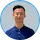Daw-Chih Liou

Daw-Chih is a software engineer, UX advocate, and creator who is dedicated to Web engineering. His background in Human Centered Computing has led him to work with startups and public companies across North America, Asia, and Europe. He is passionate about meeting business trajectory with user journey and utilizing engineering architecture and performance monitoring to provide optimal user experience.